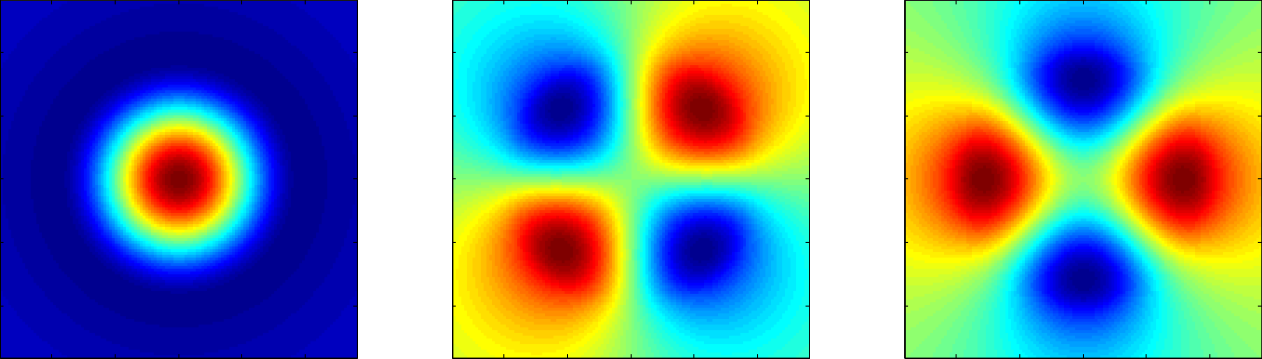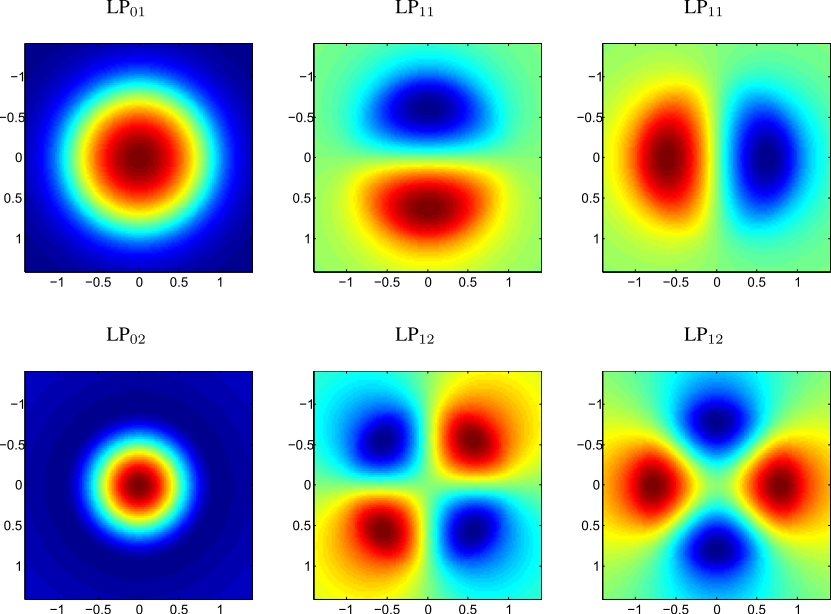# Modes of step-index multimode fibersScattering media were the first type of "complex media" for which wavefront shaping techniques were applied. Quickly, applications were developed for multimode fibers as well. One can consider multimode fiber as a complex media; because of its inherent modal dispersion (different modes travel at different speeds) and also because of the possible coupling between modes, the output field of the fiber does not resemble its input one. Wavefront shaping in multimode fibers has had a fast development because of its applications in biomedical endoscopic imaging and for telecommunications, where the exploitation of the spatial modes in multimode fibers offers a promising way to increase data rates compared to single-mode fibers.

I present here quickly the expression of the modes of a step-index multimode fiber and the so-called linearly polarized modes, that are convenient for manipulation using shaping techniques.

This post is largely inspired by Chapter 3 of [Fundamentals of Optical Waveguides] by K. Okamoto. The step-index fibers are the simplest ones for the calculation of the modes. The shapes of the modes are found analytically, the dispersion relation is analytical too but one needs numerical calculations to find its solutions.

We consider a fiber of core radius $$a$$ of refractive index $$n_1$$ surrounded by a cladding of index $$n_0$$. We assume that we satisfy in the weakly guided approximation, i.e:

$$\Delta = \frac{n_1-n_0}{n_1} \ll 1$$

In practice, this approximation is quite accurate as we have $$\Delta \leq 0.1\%$$ in standard fibers.

### Dispersion relation of Linearly Polarized (LP) modes

In the weakly guided approximation, we can define Linearly Polarized (LP) modes obtained by a combination of the TE, TM, and hybrid modes supported by the fiber that has the same propagation constant. They satisfy the general dispersion relation:

$$\frac{J_m(u)}{u J_{m-1}(u)} = \frac{K_w(u)}{w K_{m-1}(w)}$$

with $$J_m (K_m)$$ is the Bessel function of the first (second) kind of order $$m$$ and

$$u = a\sqrt{k^2 n_1^2 - \beta^2}$$
$$w = a\sqrt{\beta^2-k^2 n_0^2}$$

$$k=2\pi/\lambda$$ and $$\beta$$ is the propagation constant.

The modes are indexed by two integers $$m \geq 0$$ and $$l \geq 1$$. For a given $$m$$, the integer $$l$$ numbers the solutions of Eq. 1. For $$m = 0$$, the combination $$(m,l)$$ is two fold degenerate, for $$m \geq 1$$, the combination $$(m,l)$$ is four fold degenerate.

### Spatial profiles of the LP modes

#### TE, TM and hybrid modes

By solving the wave equation, one finds the different solutions corresponding to Transverse Electric (TE) modes, Transverse Magnetic (TE) modes, and hybrid modes (called HE and EH).

TE modes

For $$r \leq a$$:

$$E_\theta = -j \omega \mu_0 \frac{a}{u}A J_1\left(\frac{u}{a}r\right)$$
$$H_r = j \beta_l \frac{a}{u}A J_1\left(\frac{u}{a}r\right)$$
$$H_z = A J_0\left(\frac{u}{a}r\right)$$
$$E_r = E_z = H_\theta = 0$$

For $$r > a$$:

$$E_\theta = j\omega\mu_0 \frac{a}{w}\frac{J_0(u)}{K_0(w)}A K_1\left(\frac{w}{a}r\right)$$
$$H_r = -j \beta \frac{a}{w}A \frac{J_0(u)}{K_0(w)}A K_1\left(\frac{w}{a}r\right)$$
$$H_z = A\frac{J_0(u)}{K_0(w)}A K_0\left(\frac{w}{a}r\right)$$
$$E_r = E_z = H_\theta = 0$$

$$A$$ is a normalization constant.

TM modes

For $$r \leq a$$:

$$E_r = j \beta_l \frac{a}{u}A J_1\left(\frac{u}{a}r\right)$$
$$E_z = A J_0\left(\frac{u}{a}r\right)$$
$$H_\theta = j\omega \epsilon_0 n_1^2A J_1\left(\frac{u}{a}r\right)$$
$$E_\theta = H_r = H_z = 0$$

For $$r > a$$:

$$E_r = -j \beta \frac{a}{w}A \frac{J_0(u)}{K_0(w)} K_1\left(\frac{w}{a}r\right)$$
$$E_z = A\frac{J_0(u)}{K_0(w)} K_0\left(\frac{w_l}{a}r\right)$$
$$H_\theta = -j\omega \epsilon_0 n_0^2 \frac{a}{w}A \frac{J_0(u)}{K_0(w)} K_1\left(\frac{w}{a}r\right)$$
$$E_\theta = H_r = H_z = 0$$

Hybrid modes (HE and EH modes)

For $$r \leq a$$:

$$E_r = -j A \beta \frac{a}{u}\left[\frac{1-s}{2}J_{n-1}\left(\frac{u}{a}r\right)-\frac{1+s}{2}J_{n+1}\left(\frac{u}{a}r\right) \right]\cos \left(n\theta + \psi \right)$$
$$E_\theta = j A \beta \frac{a}{u} \left[\frac{1-s}{2}J_{n-1}\left(\frac{u}{a}r\right)+\frac{1+s}{2}J_{n+1}\left(\frac{u}{a}r\right) \right]\sin \left(n\theta + \psi \right)$$
$$E_z = A J_n\left(\frac{u}{a}r\right)\cos\left(n\theta + \psi\right)$$
$$H_r = -j A \omega \epsilon_0 n_1^2\frac{a}{u} \left[\frac{1-s}{2}J_{n-1}\left(\frac{u}{a}r\right)+\frac{1+s}{2}J_{n+1}\left(\frac{u}{a}r\right) \right]\sin \left(n\theta + \psi \right)$$
$$H_\theta = -j A \omega \epsilon_0 n_1^2\frac{a}{u} \left[\frac{1-s}{2}J_{n-1}\left(\frac{u}{a}r\right)-\frac{1+s}{2}J_{n+1}\left(\frac{u}{a}r\right) \right]\cos \left(n\theta + \psi \right)$$
$$H_z = -A \frac{\beta}{\omega \mu_0}s J_n\left(\frac{u}{a}r\right)\sin \left(n\theta + \psi \right)$$

For $$r > a$$:

$$E_r = -j A \beta \frac{aJ_n\left(u\right)}{wK_n\left(w\right)}\left[\frac{1-s}{2}K_{n-1}\left(\frac{w}{a}r\right)+\frac{1+s}{2}K_{n+1}\left(\frac{w}{a}r\right) \right]\cos \left(n\theta + \psi \right)$$
$$E_\theta = j A \beta \frac{aJ_n\left(u\right)}{wK_n\left(w\right)}\left[\frac{1-s}{2}K_{n-1}\left(\frac{w}{a}r\right)-\frac{1+s}{2}K_{n+1}\left(\frac{w}{a}r\right) \right]\sin \left(n\theta + \psi \right)$$
$$E_z = A \frac{J_n\left(u\right)}{K_n\left(w\right)}K_n\left(\frac{w}{a}r\right)\cos \left(n\theta + \psi \right)$$
$$H_r = -jA \omega \epsilon_0 n_0^2 \frac{aJ_n\left(u\right)}{wK_n\left(w\right)}\left[\frac{1-s}{2}K_{n-1}\left(\frac{w}{a}r\right)-\frac{1+s}{2}K_{n+1}\left(\frac{w}{a}r\right) \right]\sin \left(n\theta + \psi \right)$$
$$H_\theta = -jA \omega \epsilon_0 n_0^2 \frac{aJ_n\left(u\right)}{wK_n\left(w\right)}\left[\frac{1-s}{2}K_{n-1}\left(\frac{w}{a}r\right)+\frac{1+s}{2}K_{n+1}\left(\frac{w}{a}r\right) \right]\cos \left(n\theta + \psi \right)$$
$$H_z = - A \frac{\beta}{\omega \mu_0}s\frac{J_n\left(u\right)}{K_n\left(w\right)}K_n\left(\frac{w}{a}r\right)\sin \left(n\theta + \psi \right)$$

with $$s = \pm 1$$. By convention, we call EH modes for $$s = 1$$ and HE modes for $$s = -1$$.

### Relation between LP modes and TE, TM and hybrid modes

LP$$_\mathbf{0l}$$ modes

For $$m = 0$$, the LP modes correspond to the hybrid modes HE$$_{11}$$ with $$\psi = 0$$ and $$\psi = \pi/2$$. We then have two degenerate modes corresponding to the same mode profile but with two orthogonal linear polarizations.

$$LP_{0l} = HE_{1l}(\psi=0)$$
$$LP_{0l} = HE_{1l}(\psi=\pi/2)$$

where $$\beta = \beta_{ml}$$ obtained finding the solutions of the dispersion relation.

LP$$_\mathbf{1l}$$ modes

The linearly polarized modes for $$m = 1$$ are obtained by the superpositions of the TE mode and the HE$$_{2l}$$ mode for $$\psi = \pi/2$$ and by the superpositions of the TM mode and the HE$$_{2l}$$ mode for $$\psi = 0$$.

$$LP_{1l} = TE + HE_{2l}(\psi=\pi/2)$$
$$LP_{1l} = TE-HE_{2l}(\psi=\pi/2)$$
$$LP_{1l} = TM + HE_{2l}(\psi=0)$$
$$LP_{1l} = TM-HE_{2l}(\psi=0)$$

where $$\beta = \beta_{ml}$$ is obtained finding the solutions of the dispersion relation.

LP$$_\mathbf{ml}$$ modes, $$m > 1$$

The linearly polarized modes for $$m > 1$$ are obtained by the superposition of the hybrid modes HE$$_{m-1 l}$$ and EH$$_{m+1 l}$$ for $$\psi = 0$$ and $$\psi = \pi/2$$.

$$LP_{ml} = HE_{m+1 l}(\psi=0)+EH_{m-1 l}(\psi=0)$$
$$LP_{ml} = HE_{m+1 l}(\psi=0)-EH_{m-1 l}(\psi=0)$$
$$LP_{ml} = HE_{m+1 l}(\psi=\pi/2)+EH_{m-1 l}(\psi=\pi/2)$$
$$LP_{ml} = HE_{m+1 l}(\psi=\pi/2)-EH_{m-1 l}(\psi=\pi/2)$$

where $$\beta = \beta_{ml}$$ is obtained finding the solutions of the dispersion relation.

General expression of the LP modes

The previous expressions car be simplified using trigonometric relations to obtain a general expression for the LP modes [1,2]. For any fixed orthonormal coordinate system [X,Y,Z] with Z the propagation axis, the electric field of modes can be expressed :

LP$$_\mathbf{ml}$$

For $$r \leq a$$:

$$E_{x,y} = -j A \beta \frac{a}{u}J_{l-1}\left(\frac{u}{a}r\right) \cos \left(m\theta + \psi\right)$$
$$E_{y,x} = 0$$
$$E_z = A J_l\left(\frac{u}{a}r\right)\cos\left(m\theta + \psi\right)$$

For $$r$$ > $$a$$:

$$E_{x,y} = -j A \beta \frac{a}{u}J_{l-1}\left(\frac{u}{a}r\right) \cos \left(m\theta + \psi\right)$$
$$E_{y,x} = 0$$
$$E_z = A \frac{J_l\left(u\right)}{K_l\left(w\right)}K_l\left(\frac{w}{a}r\right)\cos \left(m\theta+ \psi\right)$$

with $$\psi$$ = 0 for m = 0 and $$\psi$$ = 0 or $$\psi = \pi$$ for m > 0 (degenerate modes).

Note that the longitudinal component is small compared to the transversal one under the weekly guided approximation.

Visual aspect of the modesFigure 1. Spatial shape of the first 6 modes.

I present if figure 1. the spatial profiles of the first four LP modes. If we define two orthogonal directions x and y orthogonal to the optical fiber axis z, for each of these figures, there exist two modes; one for which $$E_y = 0$$ and $$E_x$$ has the spatial profile represented in figure 1. and a mode where $$E_x = 0$$ and $$E_y$$ has the spatial profile of the LP mode calculated.

Created by sebastien.popoff on 27/10/2014